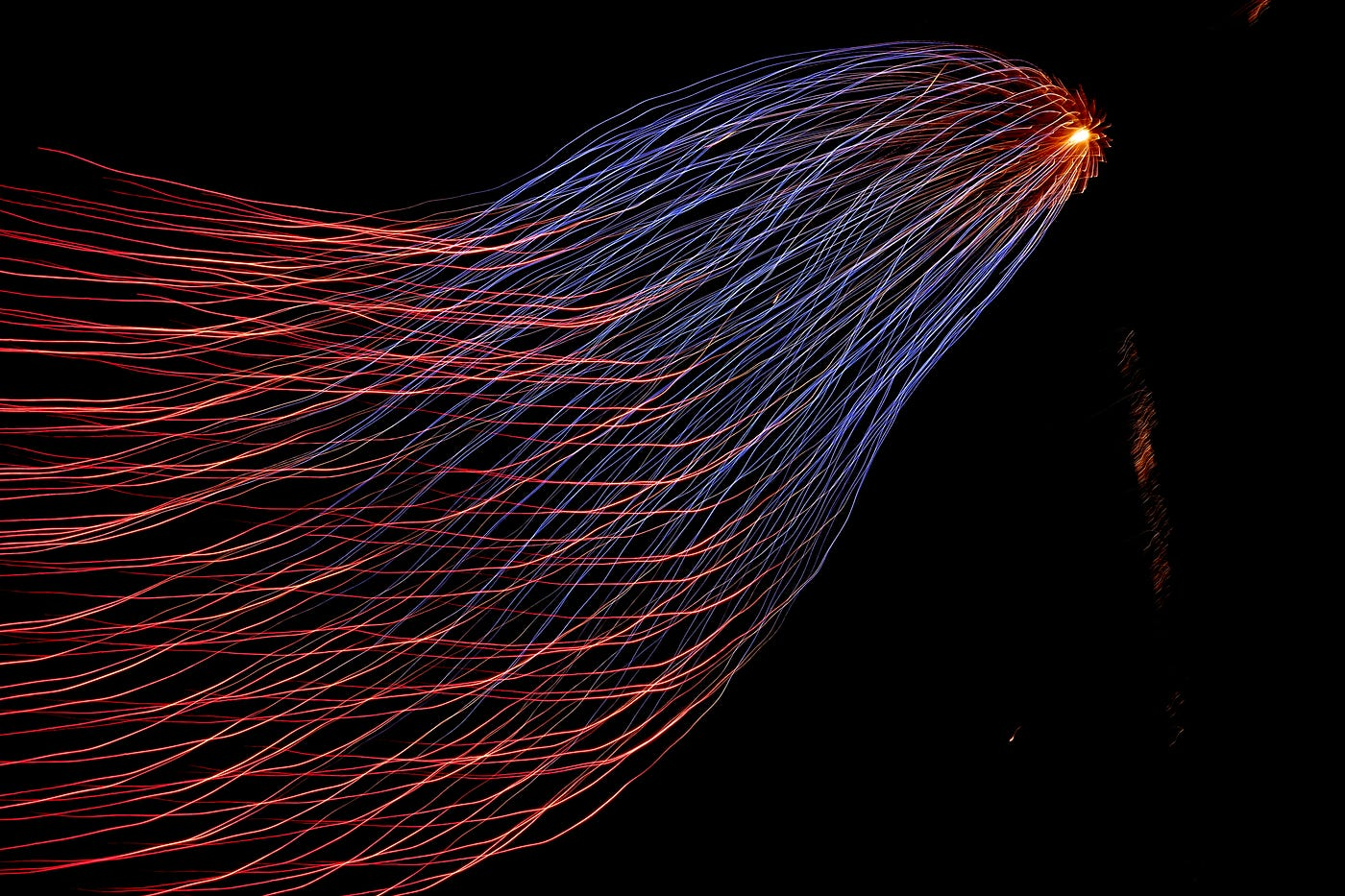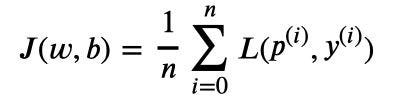# Neural Networks-A Simple IntroductionPhoto by Hunter Harritt on Unsplash

# What are Neural Networks?

Neural networks are algorithms that have a unique ability to extract meaningful information from complex data — Data that are extremely complex for a human brain to follow.

Let’s say a cat classifier, What are the features you would use to train a model to classify whether a given image is a cat or not? At first, it sounds easy, you would go for features like the size, color, paws, teeth, etc. But there are 40–70 different breeds of cats in the world and each of them differ somewhat in their color, size, etc. Now all of a sudden this became a tedious process. You can’t just manually find unique features in every single breed of cat. It would become a nightmare!

So, is there a way to extract the features from the input without doing any manual process? Absolutely!

The biggest advantage of Deep Learning is that we do not need to manually extract features from the image. The network learns to extract features while training. You just feed the image to the network (pixel values). What you need is to define the Neural Network architecture and a labeled dataset.

How do we “architect” a neural network?

# Structure of a Neural Network

A neural network can be divided into three sections

1. Input layer
2. Hidden layers
3. Output layer

## Input layer

As the name suggests itself, it is the input of our network. In our case, it is the image of a cat/non-cat.

Each node in the input layer contains a single pixel of our image. So if the dimension of the image is 64 x 64, then the total number of nodes in the input layer would be 64 x 64 x 3 = 12288 (x1, x2, . . . , x12288)

The 3 represents the three color channels of the image (Red, Green, Blue).

So now we know what the input layer is. Let’s check that off the list.

1. I̶n̶p̶u̶t̶ ̶l̶a̶y̶e̶r̶
2. Hidden layers
3. Output layer

## Hidden layers

We can have n number of hidden layers. That’s why we used the plural term layers. But for now, let’s use a single hidden layer to keep it simple to explain.

Each node in the hidden layer contains the activation value for the given combination of input values and the parameters. We know that the pixel values are the inputs. Then what are the parameters? There are 2 parameters to compute the activation function. The Weights w and the Bias b. As we can see in the image above, the input values go to all the nodes in the hidden layer, but the weights and bias they carry will be different for each node in the hidden layer.

For example, let’s consider the network in the image above. It has 3 inputs x1, x2, x3, and 4 nodes in the hidden layer a1, a2, a3, and a4. Therefore the 4 activation values will be:

a1 = σ(z1), where z1 = w1 *x1 + w2*x2 + w3*x3 + b

a2 = σ(z2), where z2 = w1 *x1 + w2*x2 + w3*x3 + b

a3 = σ(z3), where z3 = w1 *x1 + w2*x2 + w3*x3 + b

a4 = σ(z4), where z4 = w1 *x1 + w2*x2 + w3*x3 + b

Here the inputs x1, x2, and x3 will be same for all the nodes, but the weights w1, w2, w3 and the bias b will be different.

σ is called the activation function. It is the most important factor in a neural network which decides whether or not a neuron will be activated and transferred to the next layer. There are different types of activation functions, but the widely used one in the hidden layers is the ReLU activation function.

ReLu σ = max(0, z)

If z > 0, then σ(z) = z, but if z ≤ 0, then σ(z) = 0

The superscript  represents the 1st layer. The inputs x1, x2, and x3 can also be represented as a1, a2, and a3 since we don’t consider the input as a layer, like the planet Pluto, it is a planet, but not a part of the solar system. That’s not fair innit.

So now we know, that the hidden layer contains the activation values obtained by summing over the products of the weights and inputs and the bias. Let’s check that off too.

1. I̶n̶p̶u̶t̶ ̶l̶a̶y̶e̶r̶
2. H̶i̶d̶d̶e̶n̶ ̶l̶a̶y̶e̶r̶s
3. Output layer

## Output layer

For a binary classifier — in our case it’s the cat or not classifier, there will only be a single node in the output layer. The output is either 1 (cat) or 0 (not a cat).

Here, the inputs are the activation values of the hidden layer.

a2 = σ(z2), where z2 = w1*a1+ w2*a2 + w3*a3 + w4*a4 + b

The activation function σ is usually the sigmoid function in the output node for a binary classifier.

1. I̶n̶p̶u̶t̶ ̶l̶a̶y̶e̶r̶
2. H̶i̶d̶d̶e̶n̶ ̶l̶a̶y̶e̶r̶s
3. O̶u̶t̶p̶u̶t̶ ̶l̶a̶y̶e̶r̶

# Notations

Since the notations are a bit confusing above, let me explain each notation clearly

L = Total number of layers in the network.

[l] = I will use this to mention the layer of the node.

n[l] = Number of nodes in the layer l

a or x = Vector containing the input values x1, x2, . . . , xn[l]

w[l] = Matrix containing the weights of the layer l

b[l] = Vector containing the bias values of the layer l

z[l] = Vector containing the non linear values of the layer l

a[l] = Vector containing the activation values of the layer l

# Algorithm

We still haven’t seen what the neural network does to predict the output. I’ve only explained what each layer in the network contains. Now let’s see the whole algorithm.

Step 1: Forward Propagation.

Step 2: Compute the cost.

Step 3: Backward Propagation.

Step 4: Update the parameters

Step 5: Repeat until convergence of the cost.

# Forward Propagation

In forward propagation, the classifier predicts the output. The input data is fed in the forward direction through the network. Each hidden layer accepts the input data, processes it as per the activation function, and passes it to the successive layer.

z = w1*x1 + w2*x2 + w3*x3 + . . . +wn*xn[l] + b

a = σ(z)

z = w1*a1 + w2*a2 + w3*a3 + . . . + wn*an[l] + b

a = σ(z) = ypred

Here ypred is our prediction. The predictions at first will be poor since our neural network is still a baby. For that, we have a function that tells us how wrong is our fit for the weights and bias values we have currently set. The function is called the Cost function.

# Computing the cost

Usually, we train the model with a lot of labeled training examples (Different images of cats and other animals). First we need to find the loss values for each training example to compute the cost for the entire training set. Loss functions define what a good prediction is and isn’t.

Loss = -log(ypred), if y= 1

Loss = -log(1-ypred), if y= 0

After computing the loss for every training example, we’ll compute the cost. Which is nothing but the average sum of all the loss values.L(p(i), y(i)) is the loss function of the ith training example

# Backpropagation

Now we need to minimize this cost value. For that, we need the perfect combination of the parameters. Ok, of course, you can’t just manually try every combination of all possible weights and biases to find the combo that fits the best. That would take forever since you’d never run out of numbers to try.

So we use a method called gradient descent. The main purpose of this algorithm is to find the local/global minimum of a differential function. You can learn more about the cost function and the gradient descent algorithm in my previous articles.

To compute the gradient descent, we need the derivative of the parameters with respect to the loss function (dw, db, dw , db, . . . , dw[L], db[L]). So we propagate in the backward direction through the network to find the derivatives.

I will explain backpropagation in a separate article because a lot is going on in this step. But the main goal of backpropagation is to find the derivatives of the parameters with respect to the loss function.

# Gradient Descent/Updating the parameters

Proper tuning of the weights allows you to reduce error rates and to make the model reliable by increasing its generalization.

α is called the learning rate. We usually set the learning rate to be a small value, or else the cost would take a very long step and end up diverging towards the top.

Now using these new parameters we’ll repeat the whole process again and again until the cost converges and becomes closer to 0, Therefore predicting much better outputs.

# The Big Picture

Hopefully, by now you would’ve got a brief idea about neural networks. It’s okay if you didn’t understand every single bit of what I explained. Even Prof. Andrew Ng — who is a pioneer in the field of deep learning said that, at times he wouldn’t clearly understand how neural networks do what it does.

Still, there are a lot of concepts we didn’t cover in this blog since this is just an introduction. I explained a 2-Layer Neural network in this article, but there are Deep neural networks that have more than 2 hidden layers and are used for more complex problems.

The four major steps we saw in this blog is:

1. Input the image and perform forward propagation to predict the output
2. Compute the cost function to check how wrong is our prediction
3. Perform backpropagation to get the derivatives of the parameters with respect to the loss function
4. Use the derivative to perform gradient descent and update the parameters.

# Thank you!!

I hope you enjoyed it! Thank you for taking the time to read this far. If you have any suggestions, please leave a comment.

Visit my LinkedIn to know more about me and my work.

--

--